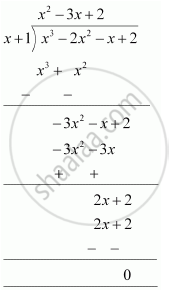# Factorise :- x^3 – 2x^2 – x + 2 - Mathematics

Factorise :- x3 – 2x2 – x + 2

#### Solution

Let p(x) = x3 − 2x2 − x + 2

All the factors of 2 have to be considered. These are ± 1, ± 2.

By trial method,

p(−1) = (−1)3 − 2(−1)2 − (−1) + 2

= −1 − 2 + 1 + 2

= 0

Therefore, (x +1) is factor of polynomial p(x).

Let us find the quotient on dividing x3 − 2x2 − x + 2 by x + 1.

By long division,It is known that,

Dividend = Divisor × Quotient + Remainder

∴ x3 − 2x2 − x + 2 = (x + 1) (x2 − 3x + 2) + 0

= (x + 1) [x2 − 2x − x + 2]

= (x + 1) [x (x − 2) − 1 (x − 2)]

= (x + 1) (x − 1) (x − 2)

= (x − 2) (x − 1) (x + 1)

Concept: Factorising the Quadratic Polynomial (Trinomial) of the type ax2 + bx + c, a ≠ 0.
Is there an error in this question or solution?
Chapter 2: Polynomials - Exercise 2.4 [Page 44]

#### APPEARS IN

NCERT Class 9 Maths
Chapter 2 Polynomials
Exercise 2.4 | Q 5.1 | Page 44

Share